# Solutions Q15 - 30#

# import all python add-ons etc that will be needed later on
%matplotlib inline
import numpy as np
import matplotlib.pyplot as plt
from sympy import *
init_printing()                      # allows printing of SymPy results in typeset maths format
plt.rcParams.update({'font.size': 16})  # set font size for plots


(a) $$I=\int\cos^3(x)dx$$. Trying $$u=\sin(x)$$, then $$du=\cos(x) dx$$ producing

$\displaystyle I=\int\cos^2(x)du=\int(1-u^2)du=u-u^3/3=\sin(x)-\sin^3(x)/2+c$

where $$c$$ is a constant and $$\cos^2+\sin^2=1$$ was used.

(b) $$\displaystyle I=\int\frac{3x}{(5+3x)^4}dx$$. Guessing $$u=5+3x$$ then $$du=dx/3$$ and substituting gives

$\displaystyle I=\int\frac{u-5}{3u^4}du =-\frac{1}{6u^2}+\frac{5}{9u^3}=-\frac{9x+5}{18(5+3x)}+c$

and eqn 6 was used.

(c) $$\displaystyle I=\int\frac{e^\sqrt{x}}{\sqrt{x}}dx$$. It seems obvious to try $$u=\sqrt{x}$$ and $$du=dx/(2\sqrt{x})$$. Substituting gives

$\displaystyle I=\int \frac{e^u}{2} du= 2e^\sqrt{x}+c$

(d) $$I=\int\cot(ax)dx$$. Recognising that $$\cot=1/\tan=\cos/\sin$$ gives $$\displaystyle I=\int\frac{\cos(x)}{\sin(x)}dx$$ and the numerator (on top) is $$1/a$$ times the derivative of $$\sin(ax)$$. Therefore, using equation 12,

$\displaystyle I=\int\cot(ax)dx = \frac{1}{a}\ln\left(\sin(ax)\right)+c$

Using Sympy as a check

x, a = symbols('x, a',positive =True)
integrate(cot(a*x),x)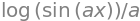(e) $$\displaystyle I=\int \frac{x^2}{8+x^3}dx$$. In this case the numerator is 1/3 of the derivative of the denominator, therefore, using equation 12,

$\displaystyle I=\int \frac{x^2}{8+x^3}dx=\frac{1}{3}\ln(8+x^3)$

(f) $$I=\int e^{ax}(1-e^{ax})^{-1}dx$$. This also has the form of equation 12 with the derivative as numerator therefore, $$I=-\ln(1-e^{ax})/a$$.

Using the substitution $$x=a\sin(u)$$ and $$dx=a\cos(u)du$$ gives

$\displaystyle I=\int\frac{1}{\sqrt{a^2-x^2}}dx=\int\frac{\cos(u)}{\sin(u)}du = u=\sin^{-1}\left( \frac{x}{a} \right)$

If a computer algebra application was used the answer $$\displaystyle \arctan\left( \frac{a}{\sqrt{a^2-x^2}} \right)$$ may be produced: $$\arctan() \equiv \tan^{-1}()$$. The inverse sine and tangents are related easily with a right-angled triangle. For example, let $$y = \sin^{-1}(x/a)$$ which is the same as writing $$\sin(y) = x/a$$. As sine is opposite / hypotenuse in a right-angled triangle, the adjacent side has length $$\sqrt{a^2 - x^2}$$ and therefore $$\tan(y)=x/\sqrt{a^2-x^2}$$ producing $$\displaystyle \sin^{-1}(x/a)=\tan^{-1}(x/\sqrt{a^2-x^2})$$Figure 41. Trig construction.

(a) Let $$u = x^2$$ and thus $$du = 2xdx$$ the integral can pleasingly be simplified to

$\displaystyle I=\frac{1}{2}\int e^{-u}du =-\frac{1}{2}e^{-u}=-\frac{1}{2}e^{-x^2} +c$

(b) Performing the definite integral

$\displaystyle I=\int_0^x xe^{-x^2}dx=-\frac{e^{-x^2}}{2}\bigg|_0^x= \frac{1-e^{-x^2}}{2} \tag{96}$

Note that this result is only valid is $$x\ge 0$$, why is this?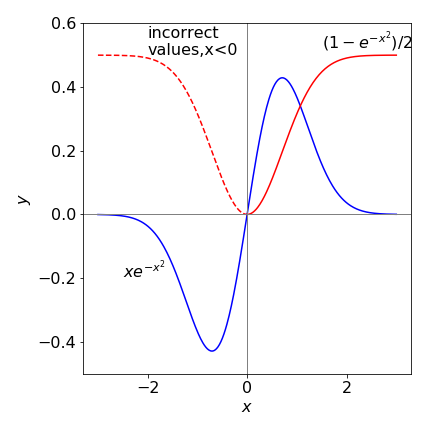Figure 42. Plot of the function $$xe^{-x^2}$$ and its integral $$(1-e^{-x^2})/2$$ vs $$x$$ (red line) showing an error for the integral when $$x \lt 0$$, dashed red line. The height of the (red) curve when $$x \ge 0$$ is the value of the integral.

Clearly the integration is incorrect when $$x \lt 0$$ because the function $$xe^{-x^2}$$ here is always negative so the integral, as the area under the curve, must also be negative. What has gone wrong? Well, it is because $$x$$ has been allowed to get smaller than the lower limit in the integral when plotting equation 96. When this happens equation 4 must be used, or the order of integration should be reversed,

$\displaystyle I=\int_{-x}^0 xe^{-x^2}dx=-\frac{e^{-x^2}}{2}\bigg|_{-x}^0= -\frac{1-e^{-x^2}}{2}$

and the only difference is a change of sign. Now this makes everything correct as shown in Figure 43 .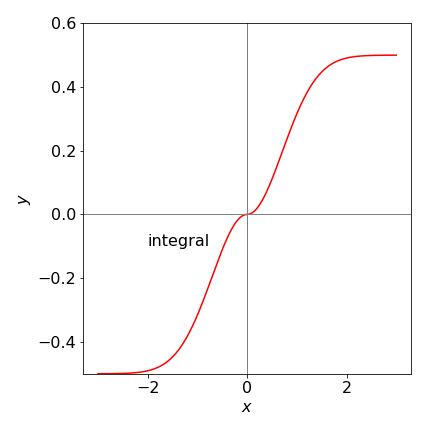Expanding the fraction gives two simpler integrals

$\displaystyle I=\int\frac{x-2}{x\sqrt{x+3}}dx=\int\frac{1}{\sqrt{x+3}}dx=-\int\frac{2}{x\sqrt{x+3}}dx$

In expressions of this form always try substituting for the square root, $$u=\sqrt{x+3}$$, then $$dy=dx/(2\sqrt{x+3})$$ and the first integral is $$I_1=\int2du=2u=2\sqrt{x+3}$$. The second is $$I_2=\displaystyle -\int\frac{2}{u^2-3}du$$, which is a standard integral, see section 2.13. The result is

$\displaystyle I_2=\frac{4}{\sqrt{3}}\tanh^{-1}\left(\frac{\sqrt{x+3}}{\sqrt{3}} \right)+c$

Some computer algebra calculations may give the $$\tanh^{-1}()$$ fraction upside down compared to this answer. In this case the two are related as $$\tanh^{-1}(w)=\tanh^{-1}(-1/w)+\pi/2$$.

Guessing at the substitution $$u = x^3$$ to get a term in $$x^2dx$$ as $$du = 3x^2dx$$. Changing the limits when $$x = -1,\, u = -1$$, and when $$x = 2, \,u = 8$$. The substituted integral can be solved by looking at the table of integrals (Section 2.13),

$\displaystyle I=\frac{1}{3}\int_{-1}^8 \frac{1}{16+u^2}du=\frac{1}{12}\tan^{-1}\left(\frac{u}{4}\right)\bigg|_{-1}^8=\frac{1}{12}\left(\tan^{-1}(2)+\tan^{-1}\left(\frac{1}{4}\right) \right)$

This integration does not look tractable, but try $$u = \sin^{-1}(ax)$$, and $$dt = dx$$ so that $$t = x$$. Evaluating gives

$\displaystyle I=\int\sin^{-1}(ax) dx=x\sin^{-1}(ax)-\int x\frac{d}{dx}\sin^{-1}(ax) dx$

Looking up or working out the deritative of $$\sin-1(ax)$$ produces $$\displaystyle I=\sin^{-1}(ax)-\int\frac{ax}{\sqrt{1-a^2x^2}}dx$$

The integral now has the form of the numerator (top) being related to the derivative of the denominator, equation 12, and the final result is

$\displaystyle I=\sin^{-1}(ax) +\frac{\sqrt{1-a^2x^2}}{a}$

(a) Changing the limits is described by equation 5, the general integral becomes

$\displaystyle \int_a^b f(x)dx=g(b)-g(a)=-\int_b^a f(x)dx$

by swapping limits and

$\displaystyle \int_{-a}^{-b} f(x)dx=g(-b)-g(-a)=\int_a^b f(-x)dx$

by negating limits.

In this particular example, negating the limit gives $$\displaystyle Ei(-x)=-\int_x^\infty \frac{e^{-t}}{t}dt$$

and swapping limits $$\displaystyle Ei(-x)=-\int_\infty^x \frac{e^{-t}}{t}dt= \int_{-\infty}^x\frac{e^t}{t}dt$$.

and the last step follows from the properties of limits, see Section 1.3.

(b) Integration by parts gives

$\displaystyle -Ei(x)= \int_{-x}^\infty \frac{e^{-t}}{t}dt=-\frac{e^{-t}}{t}\bigg|_{-x}^\infty - \int_{-x}^\infty \frac{e^{-t}}{t^2}dt = \frac{e^{x}}{x} - \int_{-x}^\infty \frac{e^{-t}}{t^2}dt$

And repeating the process the next integral is

$\displaystyle -\int_{-x}^\infty \frac{e^{-t}}{t^2}dt=\frac{e^{-t}}{t^2}\bigg|_{-x}^\infty +2 \int_{-x}^\infty \frac{e^{-t}}{t^3}dt = -\frac{e^{x}}{x^2} +2 \int_{-x}^\infty \frac{e^{-t}}{t^3}dt$

Repeating the process $$n$$ times a pattern becomes clear and is

$\displaystyle \int_{-x}^\infty \frac{e^{-t}}{t}dt=-\frac{e^{-t}}{t^n}\bigg|_{-x}^\infty -n\int_{-x}^\infty \frac{e^{-t}}{t^{n+1}}dt$

$\displaystyle -Ei(x)\approx \frac{e^x}{x} - \frac{e^x}{x^2} + 2\frac{e^x}{x^3}-6\frac{e^x}{x^4}+24\frac{e^x}{x^5} - \cdots (-1)^n n!\frac{e^x}{x^{n+1}} - \cdots n!\int_{-x}^\infty \frac{e^{-t}}{t^{n+1}}$

and the summation can be expressed as

$\displaystyle Ei(x)\approx e^x\sum_{n=0} (-1)^n\frac{n!}{x^{n+1}}$

Calculating this summation is a frustrating affair, the number produced are typically very large for example $$\approx 10^{20}$$ when $$x=50$$. The results are comparable to the answer Python/Scipy gives for large $$x$$, say, $$\pm 500$$ and for $$30$$ terms in the summation. However, with $$x \gt \approx 50$$, the results are unpredictable and vary with the number of terms in the summation. Clearly this summation is unstable and not a good way of evaluating this integral.

The instability for small $$x$$ arises because $$\pm n!/x^{n+1}$$ becomes larger and larger as $$n$$ increases and the sign alternates. This result shows how an algebraic formula, while correct, can give poor answers in a numerical calculation because of rounding errors; see Chapter 11. This is particularly true of summations with alternating positive and negative terms as in this case. Python/scipy uses the built-in function $$\mathtt{expi(x)}$$ to numerically evaluate this integral. (You need to add ‘from scipy.special import expi’ to import this function into a python worksheet).

(a) Recognize this as an integration by parts and use equation 16. If $$u = x$$ then $$dv = e^{ax}dx$$ and the formula gives

$\displaystyle \int xe^{ax}dx =x\frac{e^{ax}}{a}-\frac{1}{a}\int e^{ax} dx=\frac{(ax-1)}{a^2}e^{ax}$

(b) The result of the previous calculation produces

$I_1= xI_0 - I_0/2\quad\text{ where }\quad I_0=\int e^{ax}dx=e^{ax}/a$

Calculate the next integral by letting $$u = x$$ then $$dv = e^{ax}dx$$ and the integration by parts formula gives

$\displaystyle \int x^2 e^{ax}dx=\frac{x^2}{a}e^{ax}-\frac{2}{a}\int xe^{ax}dx$

which can be written as

$\displaystyle I_2=x^2I_0-\frac{2}{a}I_1 \tag{97}$

using $$I$$ from the question and where, logically, $$I_0$$ is defined as $$\displaystyle I_0=\int e^{ax}dx = a^{ax}/a$$. Next, define $$\displaystyle I_3=\int x^3e^{ax}dx$$ which is $$I_3=x^2I_0-3i_2/a$$ and then by induction

$\displaystyle I_n=x^nI_0-\frac{n}{a}I_{n-1} \tag{98}$

which can also be written as a recursion formula, $$aI_n+nI_{n-1}=x^ne^{ax}$$. The code below shows the recursion using Sympy. This gives the same answer as direct integration.

a, x = symbols('a, x',positive = True)

def Intn(n):
if n == 0: return exp(a*x)/a
if n == 1: return (a*x - 1)*exp(a*x)/a**2
return (x**n*exp(a*x) - n*Intn(n - 1))/a     # recursion formula
#-----------------

simplify(Intn(5) )      #  integral x^5 exp(a*x)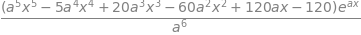(a) The integral for $$n=1$$ can be calculated between $$0$$ and infinity but the way to do this is a little cunning. The normal ‘by parts’ integral is $$\displaystyle \int u dv = uv - \int vdu$$. Now, suppose that $$\displaystyle v=e^{-ax^2}$$ therefore $$dv=-2axe^{-ax^2}$$ and also if $$u=1$$ then

$\displaystyle I_1=\frac{1}{2a}\int_0^\infty 1(-2axe^{-ax^2})dx$

which can now be integrated by parts. The $$\displaystyle -\int vdu$$ integral on the right is zero because $$u = 1$$. The result is

$\displaystyle I_1=-\frac{e^{-ax^2}}{2a}\bigg|_0^\infty -\int0dx=\frac{1}{2a}$

(b) Letting $$v = x^n$$ because $$e-x^2$$ cannot be integrated easily, gives

$\displaystyle I_n=e^{-ax^2}\frac{x^{n+1}}{n+1}\bigg|_0^\infty + 2a\int_0^\infty xe^{-ax^2}\frac{x^{n+1}}{n+1}dx$

which is

$\displaystyle I_n=e^{-ax^2}\frac{x^{n+1}}{n+1}\bigg|_0^\infty + \frac{2a}{n+1}I_{n+2}$

To work out the limits, when $$x = 0$$, then so is $$e^{ax^2}x^{n+1}$$. When $$x = \infty$$, repeated differentiation using l’Hopital’s rule produces

$\displaystyle \lim_{x\to\infty} \frac{x^{n+1}}{e^{ax^2}(n+1)}\to \frac{x^{n-1}}{2ae^{ax^2}}\to\to 0$

because for any $$n$$ the numerator will become eventually become 1, while the denominator is still infinity thus making the limit zero. Since both limits are zero then $$\displaystyle I_{n+2}=\frac{n+1}{2a}I_n$$ which is more conveniently re-written as $$\displaystyle I_{n}=\frac{n-1}{2a}I_{n-2}$$ for calculation.

The values of all other integrals of this type can now be calculated provided that $$I_0$$ is known and this has the value $$I_0 = \pi/4a$$.

a, x = symbols('a, x',positive =True)

def intxn(n):
if n == 0: return sqrt(pi/(4*a) )
if n == 1: return 1/(2*a)
if n == 2: return sqrt(pi/a)/(4*a)
return (n - 1)*intxn(n - 2)/(2*a)       # recursion chnage n to n-2
#-----------------

ans = []
for n in range( 6):   # make list to see results
ans.append(n)
ans.append(intxn(n) )
ans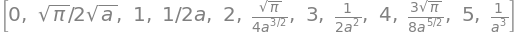The strategy here is to recognize that $$d\ln(x)=1/x$$ or, equivalently, letting $$w=\ln(x)$$ (and $$dw=dx/x$$) the integral becomes

$\displaystyle \int \frac{\cos(\ln(x))}{x}dx= \int \cos(\ln(x)) d\ln(x)\equiv\int\cos(w)dw=\sin(\ln(x))+c$

The secant function is the reciprocal of cosine and its integral is a standard one, $$\displaystyle \int \sec^2(x)dx = \tan(x)$$. The square root is removed by substitution, therefore try $$z=\sqrt{x}$$ and $$dz/dx=1/\sqrt{4x}=1/2z$$ making the integral,

$\displaystyle \int\sec^2(\sqrt{x})dx=2\int z\sec^2(z)dz$

This integral can now be tackled by the ‘parts’ method,

$\displaystyle 2\int z\sec^2(z)dz=2z\tan(z)-2\int \tan(z)dz=2z\tan(z)+2\ln(\cos(z)) +c$

where $$c$$ is a constant of integration. Substitute back for $$z$$ to get the result.

(a) This integral can be evaluated by converting to the exponential form but can also be done rather easily by parts;

$\displaystyle \int \cosh(x)\sinh(x)dx=\sinh^2(x)-\int \cosh(x)\sinh(x)dx$

and rearranging gives $$\displaystyle \int\cosh(x)\sinh(x)=\frac{1}{2}\sinh^2(x)+c$$

(b) Using the example in section 5 to evaluate the integrals gives $$\displaystyle \int x\cos(x)dx=\frac{e^x}{2}\left( \sin(x)+\cos(x) \right)$$

Converting to an exponential form gives

$\displaystyle I= \int \frac{dx}{\sin(ax)}=2i\int \frac{dx}{e^{iax}-e^{-iax}}$

which appears to have no easy solution. Try substituting $$z=e^{iax},\; dz=iae^{iax}dx$$ giving

$\displaystyle I=\frac{2}{a}\int \frac{dz}{z^2-1}$

which has a standard form, (see section 2.13) which is $$\displaystyle I= \frac{1}{2}\ln\left(\frac{z-1}{z+1} \right)$$ making the integration

$\displaystyle \int \frac{dx}{\sin(ax)}=\frac{1}{2a}\ln\left(\frac{\cos(ax)-1}{\cos(ax)+1} \right)+c$

Some texts may convert the answer into hyperbolic form using $$\displaystyle \tanh^{-1}(z)=\frac{1}{2}\ln\left( \frac{1+z}{1-z} \right)$$ where $$z$$ is defined above. In this case the result has to be real even though $$z$$ is complex, and even though the result may appear to be complex, the area under the curve, which is the integral, cannot be complex since $$1/\sin(ax)$$ is real.

(a) $$\displaystyle \int_{-\pi}^\pi dx=x\bigg|_{-\pi}^\pi =2\pi$$.

(b) Integrating and then changing the exponential to a sine using $$2i\sin(x)=e^{ix}-e^{-ix}$$ gives

$\displaystyle \int_{-\pi}^\pi e^{imx}e^{-inx}dx =-\frac{e^{-ix(m+n)}}{i(m+n)}\bigg|_{-\pi}^\pi=-\frac{ e^{-i\pi(m+n) } }{i(m+n) }+\frac{e^{i\pi(m+n)}}{i(m+n)}=2\frac{\sin\left( n\pi+m\pi \right)}{m+n}$

Recalling that $$m$$ and $$n$$ are integers let $$q = n + m$$, which can be positive, negative, or zero. Consider the case first when $$q \ne 0$$ then the integral is zero because $$\sin(\pi q) = 0$$ for any integer value of $$q$$. In the case that $$q = 0$$ then the denominator is zero and using l’Hopital’s rule (chapter 3.8) the limit is

$\lim_{q\to 0} 2\frac{\sin(q\pi}{q}\to 2\frac{\pi\cos(q\pi)}{1}\to 2\pi$

Together these results show that $$\displaystyle \int_{-\pi}^\pi e^{imx}e^{-inx}dx=2\pi\delta_{n,m}$$.

Differentiating with respect to $$\beta$$ only involves the exponential and produces

$\displaystyle \frac{d}{d\beta}\int_0^\infty \frac{e^{-\beta x}\sin(x)}{x}dx=\int_0^\infty e^{-\beta x}\sin(x)dx$

Integrating the result by parts produces

$\displaystyle \int_0^\infty e^{-\beta x}\sin(x)dx =-e^{-\beta x}\cos(x)\bigg|_0^\infty -\beta\int_0^\infty e^{-\beta x}\cos(x)dx$

Continuing the integration as in Section 5, example (c) and evaluating gives $$\displaystyle \int_0^\infty e^{-\beta x}\sin(x)dx=\frac{1}{1+\beta^2}$$. This result has to be integrated again to obtain the final result but now with respect to $$\beta$$ which is the standard integral $$\displaystyle \int\frac{d\beta }{1+\beta^2 }=\tan^{-1}(\beta)\bigg|_0^\infty =\frac{\pi}{2}$$. The final result is

$\displaystyle \int_0^\infty \frac{\sin(x)}{x}dx = \frac{\pi}{2}$

Using the definition of the cosine in a right-angled triangle, and in the limit of small values,

$\displaystyle \cos(x)=\frac{dx}{\sqrt{dx^2+dy^2}}=\left(1+\left(\frac{dy}{dx} \right)^2 \right)^{-1/2}$

then using the equation in the question $$\displaystyle 2\pi yT \left(1+\left(\frac{dy}{dx} \right)^2 \right)^{-1/2} =c$$ and rearranging to give

$\displaystyle \frac{dy}{dx}=\sqrt{\left( \frac{2\pi T}{c} \right)^2y^2-1}= \sqrt{c_0^2y^2-1}$

and, for clarity, the constants are replaced by $$c_0$$. This equation is now integrated by separating $$dy$$ and $$dx$$

$\displaystyle \int \frac{dy}{\sqrt{c_o^2y^2-1}}=\int dx=x+const$

The integral in $$y$$ evaluates to $$\displaystyle \int \frac{dy}{\sqrt{c_o^2y^2-1}}=\frac{1}{c_0}\cosh^{-1}(c_0y)$$ which produces

$\displaystyle y=\frac{\cosh(c_0x)}{c_0}$

The constants can be determined by defining the geometry of the soap film; the equation used is defined with $$y = r$$ at $$x = 0$$ therefore, $$c_0 = 1/r$$ because $$\cosh(0)=1$$